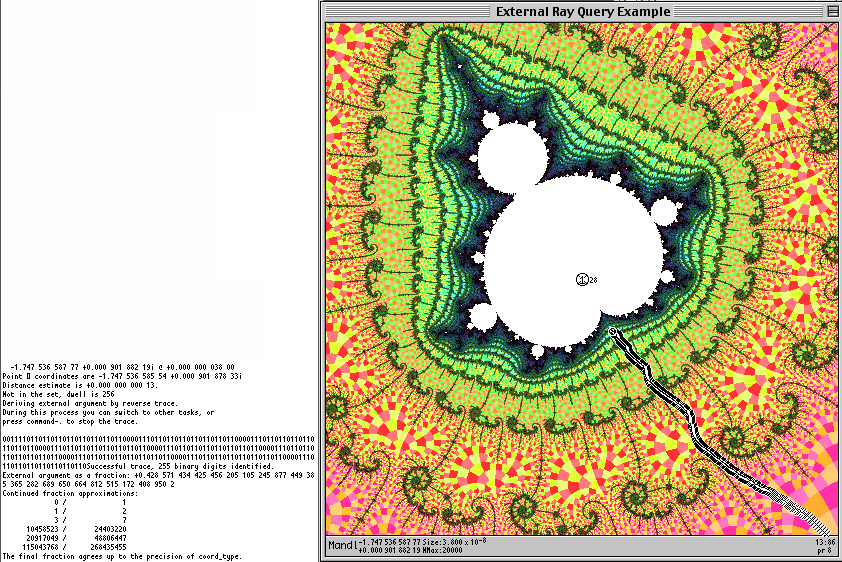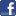# External Angle

Robert P. Munafo, 2011 Feb 14.

The external angle is a one-to-many mapping of the unit circle onto the points on the boundary of the Mandelbrot set. It provides a simple method of naming any point on the boundary. The external angle, also called external argument, is a real number between 0 and 1. The external argument multiplied by 2{pi} is the angle on the unit circle to which the boundary point has been mapped.

There are many (2c) possible continuous mappings of [0,1] onto the boundary of the Mandelbrot Set, but the one which is most useful is based on the orbit dynamics. The binary decomposition entry shows how it is computed.

If the external angle is expressed as a binary number, useful patterns can be seen. These are easy to see in an external angle plot.

### Denominators of the Form 2N-1 : External Angles of Cusps and Bond Points

If a rational external angle is expressed as a fraction A/B, the values of the numerator A and denominator B yield useful information. Of particular note, if the denominator is 2N-1, the external angle leads to the root (a bond point or cusp) of a mu-atom of period N. Every root has two external arguments except the cusp of R2 itself, which has one (although it can be expressed by two equivalent values, 0/1 and 1/1).

In this image, the program Power MANDELZOOM has computed the external angle of a point near the cusp of a period-28 island within an embedded Julia set near the cusp of R2F(1/2B1)S.Tracing an external argument

The series of continued fractions displayed by the program ends with 115043768/268435455, which is one of the external angles of the cusp of the island seen here. The fraction series also includes 3/7, which is one of the external angles of R2F(1/2B1)S (which is the influencing island of the embedded Julia set surrounding this period-28 island).

### Tracing External Rays

The above image shows the application of algorithm to trace the path to a cusp of a mu-atom of a given period N. Such algorithms typically require N bits of precision in all calculations, even when the mu-atom in question has a size much larger than 2-N; see accuracy.

### External Angles with Other Denominators

Denominators other than 2N-1 correspond to external angles that lead to branch points, tips, etc. The external angles with powers of 2 in the denominators correspond to the bifurcation in rotational symmetry of the filaments surrounding all islands. This also shows up in the symmetry of embedded Julia sets and their paramecia.

An external angle can be used as a name for the corresponding point on the Mandelbrot Set's boundary. This has an advantage over more complex naming systems in that a computer can automatically find a feature given its name. However, such names cannot be used easily by humans without the aid of external-angle software.

External angles are often used as part of a more elaborate naming system; in the R2 system they are used in the filament subset operator.

This illustration (based on the image of R2F(1/2B1)FSFSFSS) shows the "first" 32 external angles whose denominators are a power of 2.Image of R2F(1/2B1){FS(2)}x3S 3/8 5/16 1/4 3/16 '-, \ : 7/32 :- '- ~ 9/32 ' : .~ .,1/8 '`, ~, -, ` : .~ 5/32 _-' -, ", ~, ', , , :~ .- ..-` -, 11/32 'e ~, . ",a,\.`.`. .,` _-' -, ^, '\ . '. `-\$"@@LL)v'.,' . ` -, '\ ~, ~, ,`- -,,%@@F\(-' ,- ., 3/32 '-, ~, -, '. .'e'\\;"@F*`*@@/'_-` r' , _.-^ 7/16 13/32 '. _ ", '. - `:_/(,e_b@L, ./@mmL'~cm)e.-'` -,, -, ~, '- ," ', -,-]r@@*Y*"^^` '^^"*b/Y@@`"-- ,_,--''1/16 '--. -. `. .ra:c`c`.' ~//md@F^ '-:@^_^c-^' ., . -_ .`-'@@)ed@dbL`\\@*` "*@/--`` _,1/32 15/32_ '- -,-,-_:-.(@@"**^**@\$@F 'Y@^%^^^'^^'^ `-.'--`d,7/d'/dm/@F^ ^@ .@"~---- 1/2----":bLbbFb@@bmd@*^^` ' RF(2){FS}x3Sa -a/"*-'---------- 0 d;'.`"*^""-^F\$@mme ._ "mTrL___ 17/32-^` ' .~ _~-- ;^^;@@bc__.___d@, @@,m__ . - -` ,` -`(a@@@@@d@@^`\$b_ .T@Lm_ ^^^`^^--31/32 .-' ,` .,'-(@Y"[-"\```-:@@a, .a@@*C-,^` ,--` _'` ' -``/ `.-., `-\T(\$@`.,, .__a__@bF__,`^---,_ 9/16 -` /' - .' r .-'d@@@*YbFmm- -vmdbF"^@@@/"_' `^15/16 19/32 .- _~ .~ ,'-`/~)``_@b,,_a@m``;-`. -. `--. _-` .` _- ." ~.,`~TY@b@FF``-'-, `. ` _-` ./ .` -` r' -.\d@@@@c^ . ' ` , 29/32 _/ 21/32 / ." -'.'(F"\ - ` `. '-, _- .~ :~ .~ ' ` ~, -, ` , _-` .~ :- - . ~, 27/32 ' , --` a~ 23/32 .` ' -, 7/8 .- .` . 25/32 ~, 5/8 11/16 3/4 13/16

Here is a table giving the corresponding FS operators in the R2 Naming System:

 External Angle FS suffix Abbreviated FS suffix 0/1 FS FS 1/32 FS[(1/6B1)t] FS 1/16 FS[(1/5B1)t] FS 3/32 FS[(1/4B2)t] FS[4B2] 1/8 FS[(1/4B1)t] FS 5/32 FS[(1/3(1/3B1)B1)t] FS[3(3)] 3/16 FS[(1/3B2)t] FS[3B2] 7/32 FS[(1/3B1)FSFSt] FS[(3)F] 1/4 FS[(1/3B1)t] FS 9/32 FS[(1/3(2/3B1)B1)t] FS[3(2/3)] 5/16 FS[(2/5B1)t] FS[2/5] 11/32 FS[(1/2(1/4B1)B1)t] FS[2(4)] 3/8 FS[(1/2(1/3B1)B1)t] FS[2(3)] 13/32 FS[(1/2(1/2(1/3B1)B1)B1)t] FS[2(2(3))] 7/16 FS[(1/2B1)SF(1/3B1)t] FS[2SF(3)] 15/32 FS[(1/2B1)SFSF[(1/3B1)t]t] FS[(2)FS.F(3)] 1/2 FS[(1/2B1)t] FS 17/32 FS[(1/2B1)SFSF[(2/3B1)t]t] FS[(2)FS.F(2/3)] 9/16 FS[(1/2B1)SF(2/3B1)t] FS[2SF(2/3)] 19/32 FS[(1/2(1/2(2/3B1)B1)B1)t] FS[2(2(2/3))] 5/8 FS[(1/2(2/3B1)B1)t] FS[2(2/3)] 21/32 FS[(1/2(3/4B1)B1)t] FS[2(3/4)] 11/16 FS[(3/5B1)t] FS[3/5] 23/32 FS[(2/3(1/3B1)B1)t] FS[2/3(3)] 3/4 FS[(2/3B1)t] FS[2/3] 25/32 FS[(2/3B1)FSFSt] FS[(2/3)F] 13/16 FS[(2/3B2)t] FS[2/3B2] 27/32 FS[(2/3(2/3B1)B1)t] FS[2/3(2/3)] 7/8 FS[(3/4B1)t] FS[3/4] 29/32 FS[(3/4B2)t] FS[3/4B2] 15/16 FS[(4/5B1)t] FS[4/5] 31/32 FS[(5/6B1)t] FS[5/6] 1/1 FS FS

revisions: 20031218 oldest on record; 20101101 add discussion relating to cusps and bond points; 20101102 clarified wording; 20110214 add tracing section

From the Mandelbrot Set Glossary and Encyclopedia, by Robert Munafo, (c) 1987-2018.     Mu-ency index

mrob27This page was written in the "embarrassingly readable" markup language RHTF, and was last updated on 2018 Feb 04.s.11